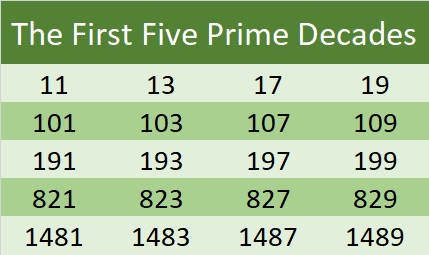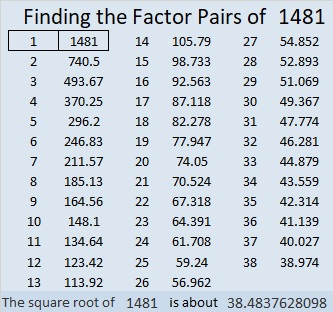# 1481 is the First Prime Number in the Fifth Prime Decade

Contents

### What Kind of Prime Number is 1481?

1481 is the 234th prime number. It is part of a twin prime pair, a prime triplet, a prime quadruplet, and even a prime quintuplet. All of those designations are nice, but to me, the most beautiful is the prime decade (the prime quadruplets not counting (5, 7, 11, 13)).1481 is the first prime number in the fifth prime decade. The last digits of the numbers in every prime decade make a lovely pattern: 1, 3, 7, and 9. Once you know the first prime number in the decade, you also know the other three! That’s beautiful!

I found the numbers in the first five prime decades easy to memorize. We start with the teen decade followed by the first and last decades in the 100’s. Then199 is almost 200 and that helps me remember 821, and 821 helps me remember 1481.

### Factors of 1481:

• 1481 is a prime number.
• Prime factorization: 1481 is prime.
• 1481 has no exponents greater than 1 in its prime factorization, so √1481 cannot be simplified.
• The exponent in the prime factorization is 1. Adding one to that exponent we get (1 + 1) = 2. Therefore 1481 has exactly 2 factors.
• The factors of 1481 are outlined with their factor pair partners in the graphic below.How do we know that 1481 is a prime number? If 1481 were not a prime number, then it would be divisible by at least one prime number less than or equal to √1481. Since 1481 cannot be divided evenly by 2, 3, 5, 7, 11, 13, 17, 19, 23, 29, 31, or 37, we know that 1481 is a prime number.

### More about the Number 1481:

1481 is the sum of two squares:
35² + 16² = 1481.

1481 is the hypotenuse of a Pythagorean triple:
969-1120-1481, calculated from 35² – 16², 2(35)(16), 35² + 16².

Here’s another way we know that 1481 is a prime number: Since its last two digits divided by 4 leave a remainder of 1, and 35² + 16² = 1481 with 35 and 16 having no common prime factors, 1481 will be prime unless it is divisible by a prime number Pythagorean triple hypotenuse less than or equal to √1481. Since 1481 is not divisible by 5, 13, 17, 29, or 37, we know that 1481 is a prime number.

This site uses Akismet to reduce spam. Learn how your comment data is processed.